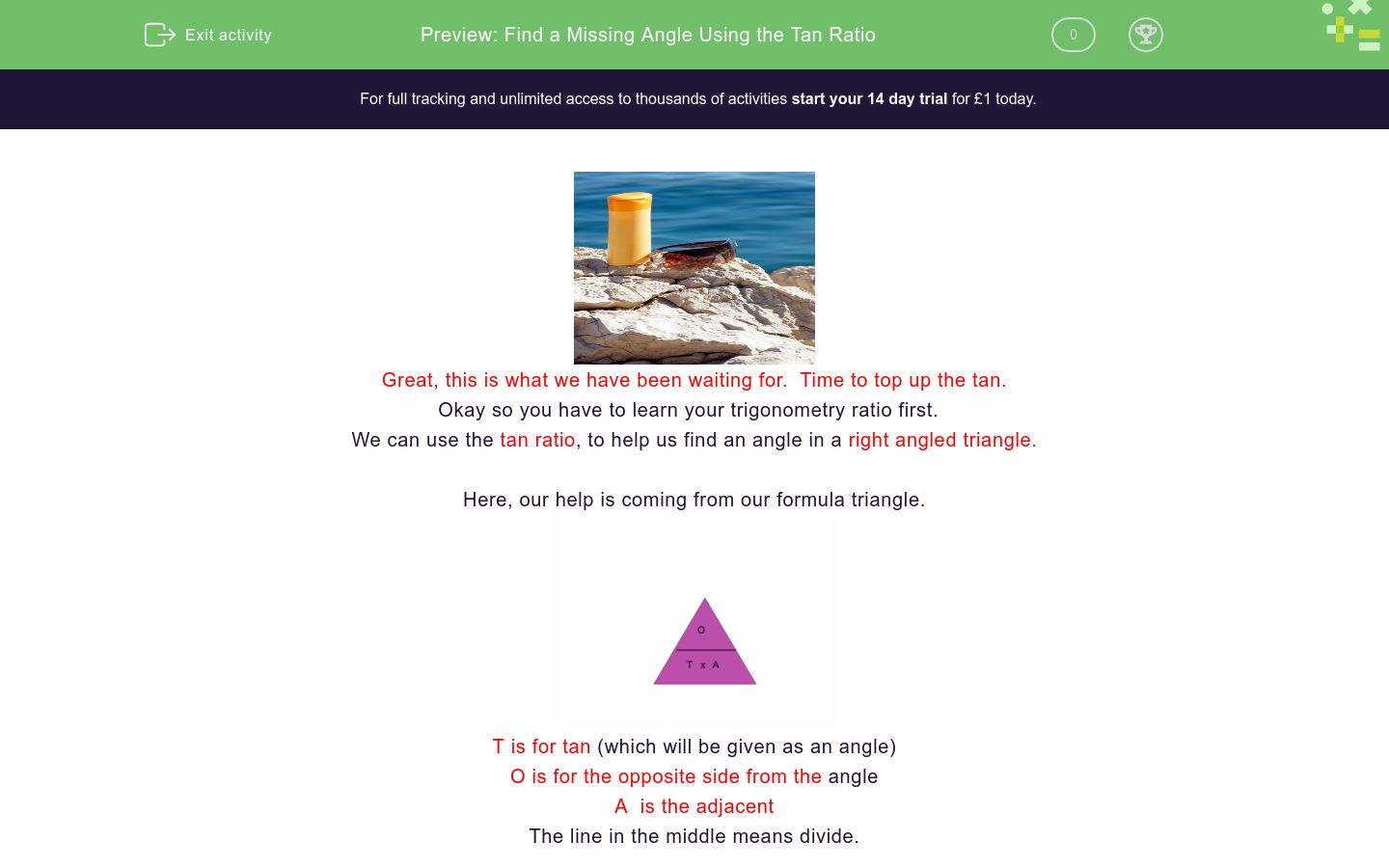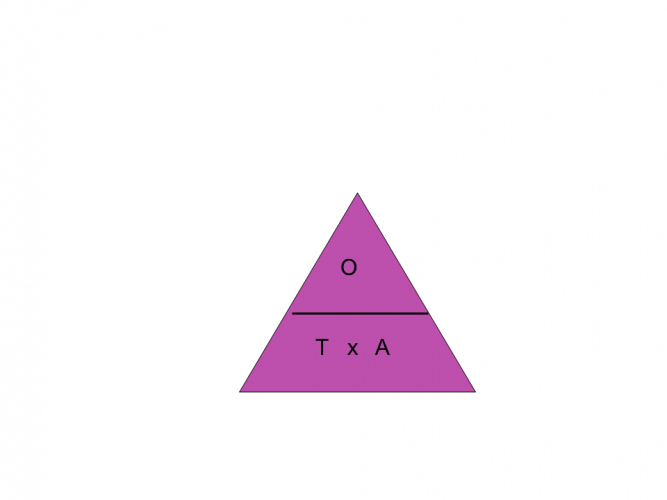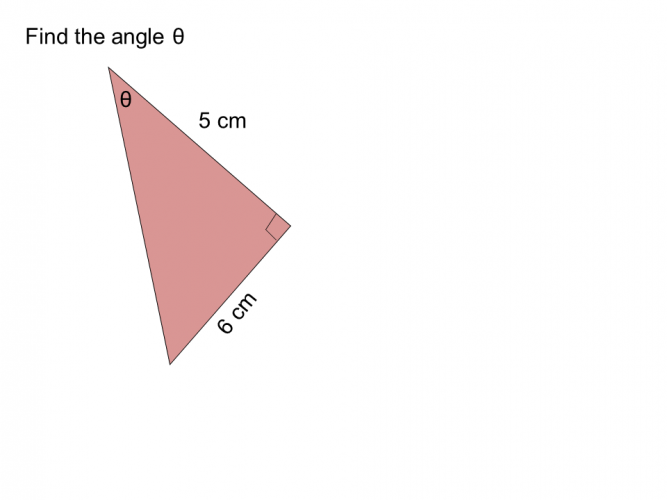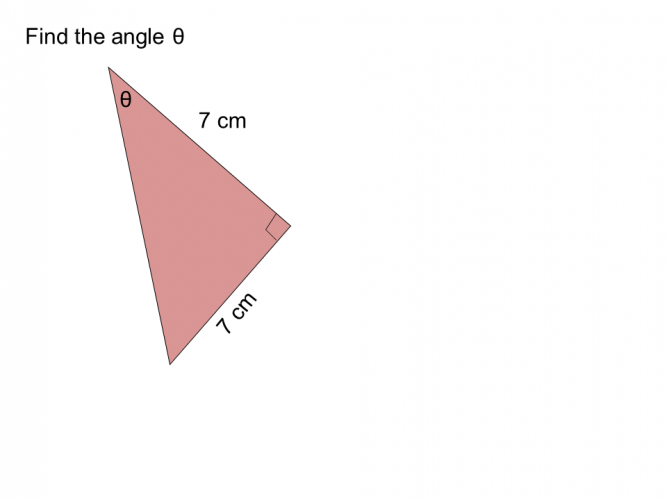# Find a Missing Angle Using the Tan Ratio

In this worksheet, students will use the Tan ratio to find an angle of a right angled triangle.Key stage:  KS 4

GCSE Subjects:   Maths

GCSE Boards:   AQA, Eduqas, Pearson Edexcel, OCR

Curriculum topic:   Geometry and Measures, Mensuration

Curriculum subtopic:   Mensuration and Calculation, Triangle Mensuration

Difficulty level:### QUESTION 1 of 10Great, this is what we have been waiting for.  Time to top up the tan.

Okay so you have to learn your trigonometry ratio first.

We can use the tan ratio, to help us find an angle in a right angled triangle.

Here, our help is coming from our formula triangle.T is for tan (which will be given as an angle)

O is for the opposite side from the angle

The line in the middle means divide.

To use this triangle we cover tan(the angle we want to find) and we are left with a formula to follow.The formula we are left with is opposite ÷ adjacent.Let's give it a go.

You will need a scientific calculator for this.  Make sure it is set to degrees.

Example 11. Label the triangle

2. Find the two sides you have to help you.  You want to find the angle, so we want O, and the only other side we have to help is the A.

3. Look at your formula triangle, cover up the T (the angle)  (see above)

4.  We are left with opposite ÷ adjacent

5. In your calculator type in  6 ÷ 5 = 1.2Here comes the geeky bit

6. To turn your 1.2 into an angle you need to access the tan-1 button on your calculator. Do this by pressing shift, then tan.

7. tan-1  1.2 = 50.2° correct to 1 decimal place.

Example 22. Find the sides you want

3.  You should have discovered you wanted O and A

4. Use your formula triangle and cover up A the angle you want to find

5. The formula you are left with is opposite ÷ adjacent

6. 3 ÷ 2 = 1.5

7. Do the geeky bit, tan-1 1.5 = 56.3° to 1 decimal place.

How tantastic is that?What is the size of the angle marked?

Answer has been rounded to 1 decimal place.

20.9°

23.4°

22.5°

21.8°tan ÷ adjacent opposite ÷ adjacent 45° 55° 65° The formula I need is The angle istan ÷ adjacent opposite ÷ adjacent 45° 55° 65° The formula I need is The angle is46.20°

66.03°

55.18°

70.01°36.47° 43.41° 45.0° 38.62° The angle is36.47° 43.41° 45.0° 38.62° The angle isAnswer has been rounded to 1 decimal place (1 d.p)

59.9°

61.0°

58.9°

63.2°

• Question 1What is the size of the angle marked?

Answer has been rounded to 1 decimal place.

21.8°
EDDIE SAYS
Labelling your triangle correctly can be tantalisingly tricky. You should have discovered that you only have the opposite and adjacent sides to help, so our formula is opposite ÷ adjacent 4 ÷ 10 = 0.4 Do the geeky bit tan-1 0.4 = 21.8° (to 1 d.p)
• Question 250.2
EDDIE SAYS
When you label your triangle you will only find the two sides you need to help. The two letters (e.g O and A)only appear in the tan formula triangle, so you know which one to use. 6 ÷5 = 1.2 tan-1 1.2 = 50.2° ( to 1 d.p)
• Question 3tan ÷ adjacent opposite ÷ adjacent 45° 55° 65° The formula I need is The angle is
EDDIE SAYS
I hope you are not getting tan(gled) up with this all. It soon becomes second nature with practice. 7 ÷ 7 = 1 tan-1 1= 45°
• Question 4EDDIE SAYS
Have you notice that when you need to find an angle, the formula is always the same, unlike when trying to find a side. So once you know the formula it makes things a little easier. O ÷ A 8 ÷ 11 = 0.7272 tan-1 0.7272 = 36.03°
• Question 566.03°
EDDIE SAYS
Are you getting used to this now? We want the angle so the formula is O ÷ A as normal 9 ÷ 4 = 2.25 tan-1 2.25 = 66.03° to (2 d.p).
• Question 624.44
EDDIE SAYS
I hope you remember that the formula is always O ÷A. It can look awkward sometimes as we are generally used to dividing a larger number by a smaller number. Don't worry, always trust your formula. 2.5 ÷ 5.5 = 0.4545 tan-1 0.4545 = 24.44° (to 2 d.p)
• Question 736.47° 43.41° 45.0° 38.62° The angle is
EDDIE SAYS
I hope you are getting the hang of this now and not having tan trums. 8.5 ÷ 11.5 =0.739... tan-1 0.739..= 36.47°
• Question 8EDDIE SAYS
I hope you are getting on well here and not going off on a tangent. Keeping on track gives us 8 ÷ 6 = 1.333 tan-1 1.333 =53.13 (to 2 d.p)
• Question 966.04
EDDIE SAYS
Keep going, practice makes perfect. 9 ÷ 4 = 2.25 tan-1 2.25 = 66.04° (to 2 d.p)
• Question 10Answer has been rounded to 1 decimal place (1 d.p)

59.9°
EDDIE SAYS
Nearly time to check out and top up those sun tans One final check though 19 ÷ 11 = 1.7272.... tan-1 1.727272 = 59.9° I hope everything is tantastic.
---- OR ----

Sign up for a £1 trial so you can track and measure your child's progress on this activity.

### What is EdPlace?

We're your National Curriculum aligned online education content provider helping each child succeed in English, maths and science from year 1 to GCSE. With an EdPlace account you’ll be able to track and measure progress, helping each child achieve their best. We build confidence and attainment by personalising each child’s learning at a level that suits them.

Get started